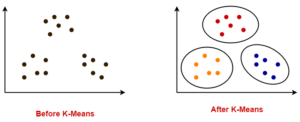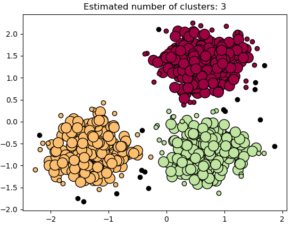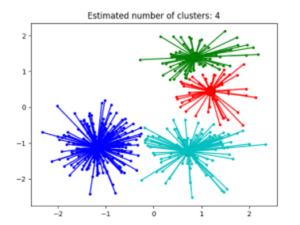Select Page

What is Clustering?

It is basically a type of unsupervised machine learning method . An unsupervised learning method is a method in which we draw references from datasets consisting of input data without labelled responses. Data clustering is an effective method for data analysis and pattern recognition which has been applied in many fields such as image segmentation, machine learning and data mining. It is the process of splitting the multidimensional data into several groupings or clusters based on some similarity measures. A cluster is usually defined by a cluster center. We use a type of clustering algorithm where the complete data is viewed as a network with each data point being a node in the network.

Clustering Methods :

• Centroid Clustering
• Density Based Clustering
• Distribution Clustering
• Connectivity Clustering

Centroid Clustering:

In this type of clustering method, for obtaining the clusters firstly we have to define the number of clusters required and also tell the number of points inside the cluster including the distance. The example of Centroid clustering is the K-Means algorithm, where K denotes the number of clusters. The centroid clustering algorithms are best when we initially know the exact number of clusters, although K-means is very popular and simple to use.Density Based Clustering:

The Density Based Clustering is used when we have densely based data points, in this type of clustering method we dont require to mention or to predict the exact number of predefined clusters. The Density based clustering algorithms will automatically figure out the numbers of clusters. The Density based clustering algorithms are DBSCAN and OPTICS. These algortihms are very popular because they deal very well  with outliers data points.

The DBSCAN algorithm only accepts two input parameters that are eps. and minPts and they will return the clusters formed by the algorithm. The OPTICS algorithm is more efficient than DBSCAN, it requires only  minPts in each cluster, and then it will return the final formed clusters.Distribution Clustering:

Distributed  clustering is widely used in the last decade because they deal with very large and heterogeneous datasets which cannot be gathered centrally. The example of Distributed  clustering is Mean Shift clustering. It works very well because it’s like the probability of one data point belonging to this cluster versus that point definitely belonging to this cluster.Connectivity Clustering:

The clusters formed in this method forms a tree-type structure based on the hierarchy. New clusters are formed using the previously formed one. It is divided into two categories that are Agglomerative (bottom up approach) and Divisive (top down approach). The example of connectivity cluterings are Affinity Propagation and Agglomerative Clustering.